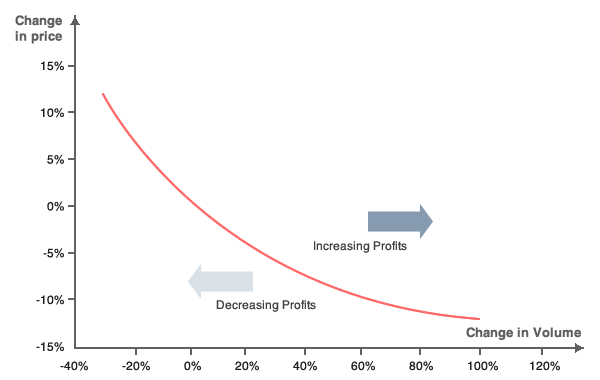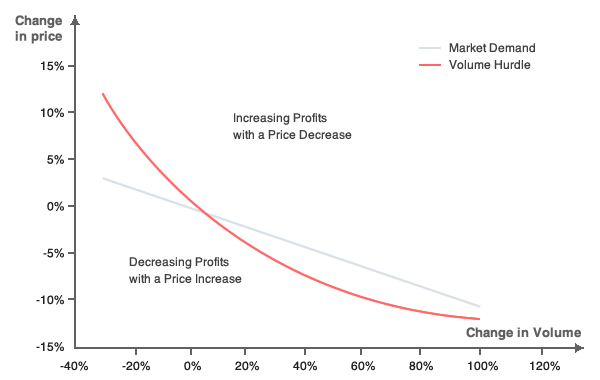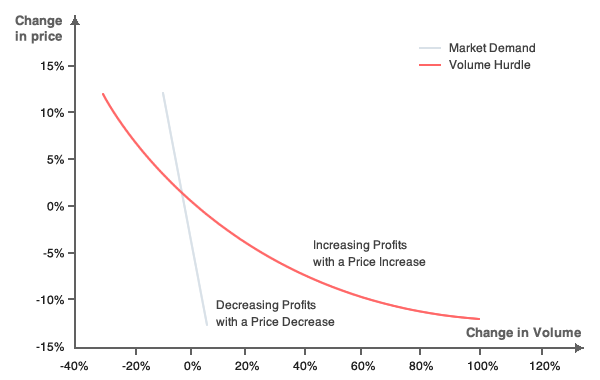# Price Elasticity of DemandBy Gaël Grasset, July 2015

In modern microeconomics, price elasticity measures the correlation between the variation in demand and the variation in price. If the market is elastic, a tiny change in price results in a large change in sales volume. If the market is inelastic, in this case, a large change is price results in a tiny change in sales volume. Therefore, the pricing strategy will be different depending on whether the market is either elastic or inelastic:

• If the market is elastic, increasing profits implies decreasing the price.
• If the market is inelastic, increasing profits implies increasing the price.

## Using elasticity in pricing

The following iso-profit curve illustrates the relationship between variations in price and variations in sales volume while retaining a constant 25% profit margin:The price per unit is $10 with a 25% margin; therefore the cost per unit is$7.5. The initial profit for selling 100 units is \$250. With a 12 % decrease in price, the firm now needs to sell 200 units (+100% of sales volume) in order to make the same profit. This is how we define iso-profit.

### Elastic markets

In elastic markets, changes in price result in demand volatility. Therefore, the best pricing strategy is to decrease the price in order to sell much more of a given product. This is demonstrated by the two curves in the diagram below. The initial position is at (0%; 0%); the iso-profit curve cuts the demand curve at this point. All the points on the demand curve which are above the iso-profit curve demonstrate an increase in profit. Therefore, we can establish that the firm in question can increase its profits by decreasing the price.For example, according to Ayers and Collinge, the demand for soda (Coca-Cola or Mountain Dew) is very elastic. This means that a small variation in price could produce a large change in the demand, which comes from the competition that exists in the soda market. If Coca-Cola becomes more expensive, consumers will, to some extent, prefer to buy Pepsi, and therefore the demand for Coca-Cola will collapse. On the other hand, Coca-Cola is aware of the demand elasticity for its products and could indeed decide to cut the price of its drink, thereby decreasing the demand for Pepsi.

### Inelastic markets

In inelastic markets, demand is virtually independent of variations in price. Therefore, the best pricing strategy in this case is to increase the price in order to sell the same quantity as before (i.e. prior to the price increase), and to maximize profit margins as a result. This is demonstrated by the diagram below. The initial position is at (0%; 0%); the iso-profit curve cuts the demand curve at this point. All the points on the demand curve which are above the iso-profit curve increase the profit. Therefore, we can see that the firm in question can increase its profits by increasing the price.For example, according to Havranek et al, the demand for gasoline (either in the short-term or the long-term) is inelastic. This means that a large variation in price produces a small change in the demand. If Gasoline becomes more expansive, the demand will pay for it. People can’t change their habit on the short term, they need their cars in order to go to work for example.

## Elasticity in economic theory

Elasticity is calculated with the following formula:

$$E_d = \frac{\frac{dQ}{Q}}{\frac{dP}{P}}$$

It measures the sensitivity of the quantity demanded ($$Q$$) according to changes in the price ($$P$$). Elasticity is almost always negative as the relationship between the demand and the price is decreasing in most situations. To illustrate: an increase in price results in a decrease in demand in the vast majority of cases. The value of elasticity indicates to what extend a firm can experiment with different pricing methods. For example, if the demand its elastic, the costs resulting from changing the pricing could be huge. On the contrary, if the demand is inelastic, setting a higher price could significantly increase profits; so an increase in price is indeed more effective in this instance.

Value Interpretation Example How to increase profit?
$$E_d>0$$ Veblen or Giffen1 Highly distinctive products such as in the fashion industry Increase price
$$E_d=0$$ Perfect inelasticity Insulin for diabetics Increase price
$$-1<E_d<0$$ Relative inelastic demande Gasoline Increase price
$$E_d=-1$$ Unit elasticity Wine in the USA Nothing to do
$$-∞<E_d<-1$$ Relative elastic demand Coca-Cola Decrease price
$$E_d=-∞$$ Perfect elastic demand Highly commpetitive goods : potatos on supermarket Decrease price

In order to determine the most effective pricing method to use, it is first necessary to establish whether a market is elastic or inelastic, which will indicate whether it is best to increase or decrease the price.

## Long-term and short-term elasticity

The concept of elasticity was introduced by Alfred Marshall as a short-term concept used for modeling the short-term impact of shifts in the price structure. That being said, shifts in pricing can actually have more impact in the long-term than in the short-term. For example, if the price of gasoline is multiplied by 20, in the short-term, everybody will continue paying for gasoline for the first few years. However, electric motors will eventually be developed and technology will evolve in order to put an end to the use of gasoline. Consequently, the maximization of profit in the short-term could be an incentive for other firms to find new substitutable goods, thereby decreasing the profit in the long-term.

• Havranek T., Irsova Z. & Janda K., “Demand for gasoline is more price-inelastic than commonly thought”, Energy economics, 2012
• Marshall A., Principles of economics, 1890
• Smith T. J., Pricing Strategy. Setting price levels, managing price discounts & establishing price structures, 2012

1. Veblen goods are distinctive product, customers buy it in order to show their wealth and therefore the demand increase when the price increase. Giffen goods are goods of primary necessity such as food. If the price of food increase, the demande increases too meaning that a larger part of budget will be dedicated to this. ↩︎